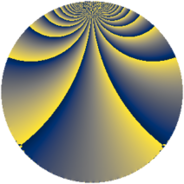# Properties

 Label 684.3.bxLevel $684$ Weight $3$ Character orbit 684.bx Rep. character $\chi_{684}(109,\cdot)$ Character field $\Q(\zeta_{18})$ Dimension $102$ Sturm bound $360$

# Related objects

## Defining parameters

 Level: $$N$$ $$=$$ $$684 = 2^{2} \cdot 3^{2} \cdot 19$$ Weight: $$k$$ $$=$$ $$3$$ Character orbit: $$[\chi]$$ $$=$$ 684.bx (of order $$18$$ and degree $$6$$) Character conductor: $$\operatorname{cond}(\chi)$$ $$=$$ $$19$$ Character field: $$\Q(\zeta_{18})$$ Sturm bound: $$360$$

## Dimensions

The following table gives the dimensions of various subspaces of $$M_{3}(684, [\chi])$$.

Total New Old
Modular forms 1512 102 1410
Cusp forms 1368 102 1266
Eisenstein series 144 0 144

## Trace form

 $$102q + 9q^{7} + O(q^{10})$$ $$102q + 9q^{7} - 15q^{11} + 9q^{13} + 9q^{17} + 12q^{19} - 96q^{23} + 18q^{25} - 93q^{29} - 108q^{31} - 111q^{35} + 18q^{41} + 117q^{43} + 15q^{47} - 342q^{49} - 75q^{53} - 3q^{55} + 165q^{59} + 132q^{61} + 225q^{65} + 531q^{67} + 90q^{71} - 90q^{73} + 108q^{77} + 39q^{79} + 207q^{83} - 234q^{85} + 168q^{89} + 402q^{91} + 393q^{95} + 279q^{97} + O(q^{100})$$

## Decomposition of $$S_{3}^{\mathrm{new}}(684, [\chi])$$ into newform subspaces

The newforms in this space have not yet been added to the LMFDB.

## Decomposition of $$S_{3}^{\mathrm{old}}(684, [\chi])$$ into lower level spaces

$$S_{3}^{\mathrm{old}}(684, [\chi]) \cong$$ $$S_{3}^{\mathrm{new}}(19, [\chi])$$$$^{\oplus 9}$$$$\oplus$$$$S_{3}^{\mathrm{new}}(38, [\chi])$$$$^{\oplus 6}$$$$\oplus$$$$S_{3}^{\mathrm{new}}(57, [\chi])$$$$^{\oplus 6}$$$$\oplus$$$$S_{3}^{\mathrm{new}}(76, [\chi])$$$$^{\oplus 3}$$$$\oplus$$$$S_{3}^{\mathrm{new}}(114, [\chi])$$$$^{\oplus 4}$$$$\oplus$$$$S_{3}^{\mathrm{new}}(171, [\chi])$$$$^{\oplus 3}$$$$\oplus$$$$S_{3}^{\mathrm{new}}(228, [\chi])$$$$^{\oplus 2}$$$$\oplus$$$$S_{3}^{\mathrm{new}}(342, [\chi])$$$$^{\oplus 2}$$